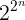# Minimizing random Boolean expressions

The previous post looked at all Boolean expressions on three or four variables and how much they can be simplified. The number of Boolean expressions on n variables isand so the possibilities explode as n increases. We could do n = 3 and 4, but 5 would be a lot of work, and 6 is out of the question.

So we do what we always do when a space is too big to explore exhaustively: we explore at random.

The Python module we’ve been using, `qm`, specifies a function of n Boolean variables in terms of the set of product terms on which the function evaluates to 1. These product terms can be encoded as integers, and so a Boolean function of n variables corresponds to a subset of the integers 0 through 2n – 1.

We can generate a subset of these numbers by generating a random mask consisting of 0s and 1s, and keeping the numbers where the mask value is 1. We could do this with code like the following.

```     N= 2**n
x = np.arange(N)
```

There’s a small problem with this approach: the set `ones` always contains 0. We want it to contain 0 if and only if the 0th mask value is a 1.

The following code generates a Boolean expression on n variables, simplifies it, and returns the length of the simplified expression .

```    def random_sample(n):
N = 2**n
x = np.arange(N)
ones.remove(0)
return len(qm(ones=ones, dc={}))
```

We can create several random samples and make a histogram with the following code.

```    def histogram(n, reps):
counts = np.zeros(2**n+1, dtype=int)
for _ in range(reps):
counts[random_sample(n)] += 1
return counts
```

The data in the following graph comes from calling `histogram(5, 1000)`.Note that the length of the random expressions is distributed symmetrically around 16 (half of 25). So minimization turns a distribution centered around 16 into a distribution centered around 8.

The code is slow because the Quine-McCluskey algorithm is slow, and our Python implementation of the algorithm isn’t as fast as it could be. But Boolean minimization is an NP problem, so no exact algorithm is going to scale well. To get faster results, we could switch to something like the Expresso Heuristic Logic Minimizer, which often gets close to a minimum expression.

***

 The code above will fail if the set of terms where the function is 1 is empty. However this is extremely unlikely: we’d expect it to happen once in every 2^(2^n) times and so when n = 5 this is less than one time in four billion. The fully correct approach would be to call `qm` with `zeros=x` when `ones` is empty.